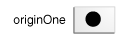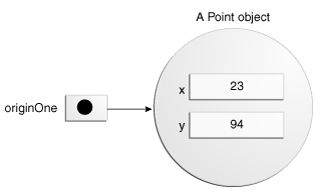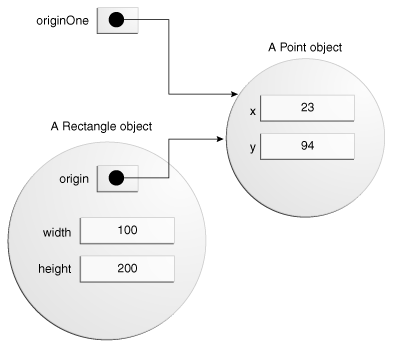Documentation

The Java™ Tutorials
Hide TOC
Trail: Learning the Java Language
Lesson: Classes and Objects
Section: Objects

# Creating Objects

As you know, a class provides the blueprint for objects; you create an object from a class. Each of the following statements taken from the `CreateObjectDemo` program creates an object and assigns it to a variable:

```Point originOne = new Point(23, 94);
Rectangle rectOne = new Rectangle(originOne, 100, 200);
Rectangle rectTwo = new Rectangle(50, 100);
```

The first line creates an object of the `Point` class, and the second and third lines each create an object of the `Rectangle` class.

Each of these statements has three parts (discussed in detail below):

1. Declaration: The code set in bold are all variable declarations that associate a variable name with an object type.
2. Instantiation: The new keyword is a Java operator that creates the object.
3. Initialization: The new operator is followed by a call to a constructor, which initializes the new object.

## Declaring a Variable to Refer to an Object

Previously, you learned that to declare a variable, you write:

```type name;
```

This notifies the compiler that you will use name to refer to data whose type is type. With a primitive variable, this declaration also reserves the proper amount of memory for the variable.

You can also declare a reference variable on its own line. For example:

```Point originOne;
```

If you declare `originOne` like this, its value will be undetermined until an object is actually created and assigned to it. Simply declaring a reference variable does not create an object. For that, you need to use the `new` operator, as described in the next section. You must assign an object to `originOne` before you use it in your code. Otherwise, you will get a compiler error.

A variable in this state, which currently references no object, can be illustrated as follows (the variable name, `originOne`, plus a reference pointing to nothing):## Instantiating a Class

The new operator instantiates a class by allocating memory for a new object and returning a reference to that memory. The new operator also invokes the object constructor.

Note: The phrase "instantiating a class" means the same thing as "creating an object." When you create an object, you are creating an "instance" of a class, therefore "instantiating" a class.

The new operator requires a single, postfix argument: a call to a constructor. The name of the constructor provides the name of the class to instantiate.

The new operator returns a reference to the object it created. This reference is usually assigned to a variable of the appropriate type, like:

````Point originOne = new Point(23, 94);`
```

The reference returned by the new operator does not have to be assigned to a variable. It can also be used directly in an expression. For example:

```int height = new Rectangle().height;
```

This statement will be discussed in the next section.

## Initializing an Object

Here's the code for the Point class:

```public class Point {
public int x = 0;
public int y = 0;
//constructor
public Point(int a, int b) {
x = a;
y = b;
}
}
```

This class contains a single constructor. You can recognize a constructor because its declaration uses the same name as the class and it has no return type. The constructor in the Point class takes two integer arguments, as declared by the code (int a, int b). The following statement provides 23 and 94 as values for those arguments:

```Point originOne = new Point(23, 94);
```

The result of executing this statement can be illustrated in the next figure:Here's the code for the Rectangle class, which contains four constructors:

```public class Rectangle {
public int width = 0;
public int height = 0;
public Point origin;

// four constructors
public Rectangle() {
origin = new Point(0, 0);
}
public Rectangle(Point p) {
origin = p;
}
public Rectangle(int w, int h) {
origin = new Point(0, 0);
width = w;
height = h;
}
public Rectangle(Point p, int w, int h) {
origin = p;
width = w;
height = h;
}

// a method for moving the rectangle
public void move(int x, int y) {
origin.x = x;
origin.y = y;
}

// a method for computing the area of the rectangle
public int getArea() {
return width * height;
}
}

```

Each constructor lets you provide initial values for the rectangle's origin, width, and height, using both primitive and reference types. If a class has multiple constructors, they must have different signatures. The Java compiler differentiates the constructors based on the number and the type of the arguments. When the Java compiler encounters the following code, it knows to call the constructor in the Rectangle class that requires a Point argument followed by two integer arguments:

```
Rectangle rectOne = new Rectangle(originOne, 100, 200);
```

This calls one of `Rectangle`'s constructors that initializes `origin` to `originOne`. Also, the constructor sets `width` to 100 and `height` to 200. Now there are two references to the same Point object—an object can have multiple references to it, as shown in the next figure:The following line of code calls the `Rectangle` constructor that requires two integer arguments, which provide the initial values for width and height. If you inspect the code within the constructor, you will see that it creates a new Point object whose x and y values are initialized to 0:

```Rectangle rectTwo = new Rectangle(50, 100);
```

The Rectangle constructor used in the following statement doesn't take any arguments, so it's called a no-argument constructor:

```Rectangle rect = new Rectangle();
```

All classes have at least one constructor. If a class does not explicitly declare any, the Java compiler automatically provides a no-argument constructor, called the default constructor. This default constructor calls the class parent's no-argument constructor, or the `Object` constructor if the class has no other parent. If the parent has no constructor (`Object` does have one), the compiler will reject the program.

Previous page: Objects
Next page: Using Objects